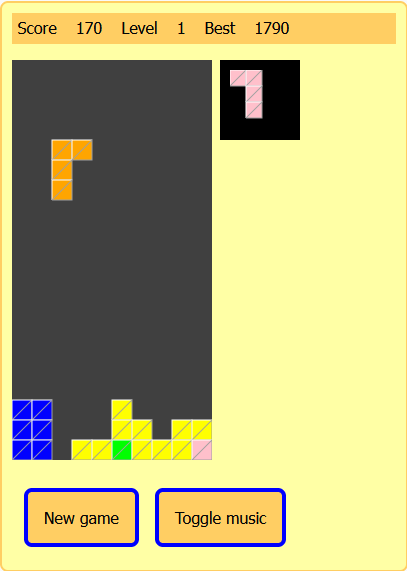## Tetris is back online at Arcade Village

2022-02-04 Site life
Tetris was in the jukebox, but I bragged that I could switch it from java to javascript in two days. Bet won!

So you can play this version online now.## If you like programming

It was easy to win my bet to make this game in javascript in two days (I even added sounds compared to the original version), because I had already written a version in java and the use of classes facilitates the passage between the two languages.
Here is the class "element" used in the game is written, first in java, then in javascript.

### The java version

``` package tetris; import java.awt.*; public class element { int ia = 0; // Actual apparence index apparence aa; // Actual apprence object Dimension rd; Point pos; // Position, in rectangle value of the left-up corner public Color c; int ci; public element( int cx, int cy ) { this.rd = new Dimension(cx,cy); } public void rotateLeft() { ia--; if ( ia < 0 ) ia = 3; } public void rotateRight() { ia++; if ( ia > 3 ) ia = 0; } /** * * Paint the element with the default rectangle dimension * */ public void paint( Graphics g ) { paint(g,pos,rd.width,rd.height); } /** * * Paint the element with a specified rectangle dimension * */ public void paint( Graphics g, Point pos, int cx, int cy ) { if ( pos == null ) return; Point p = new Point(pos); for ( int y = 0 ; y < aa.ty ; y++ ) { for ( int x = 0; x < aa.tx ; x++ ) { if ( aa.getValue(ia,x,y) ) // Does we have to draw a rectangle { // yes ! drawCase( g,c, p.x, p.y, cx, cy ); } p.x += 1; } p.y += 1; p.x = pos.x; } } public static void drawCase( Graphics g, Color c, int ax, int ay, int cx, int cy ) { int x = ax * cx; int y = ay * cy; Polygon pl; g.setColor( c ); g.fillRect(x,y, cx,cy ); pl = new Polygon(); g.setColor(Color.white); pl.addPoint(x, y + cy ); pl.addPoint(x, y); pl.addPoint(x + cx, y); g.drawPolygon(pl); g.setColor( c.darker() ); pl = new Polygon(); pl.addPoint(x, y + cy ); pl.addPoint(x + cx, y + cy ); pl.addPoint(x + cx, y); pl.addPoint(x, y + cy ); g.drawPolygon(pl); } public boolean touch( int[] is, int ncx, int ncy ) { return aa.touch(ia, is, pos.x, pos.y, ncx, ncy); } } ```

### The translated version in javascript

``` class element { constructor( cx, cy ) { this.rd_width = cx; this.rd_height = cy; this.ia = 0; // Actual apparence index this.aa = null; // Actual apprence object this.posx = 0; // Position, in rectangle value of the left-up corner this.posy = 0; // Position, in rectangle value of the left-up corner this.width = 0; this.height = 0; this.c = ""; // Color this.ci = 0; } rotateLeft() { this.ia--; if ( this.ia < 0 ) this.ia = 3; } rotateRight() { this.ia++; if ( this.ia > 3 ) this.ia = 0; } setParameters( apparence, posx, posy, couleur ) { this.aa = apparence; this.posx = posx; this.posy = posy; this.c = couleur; } touch( is, ncx, ncy ) { return this.aa.touch( this.ia, is, this.posx, this.posy, ncx, ncy); } /** * * Pathe element with the default rectangle dimension * */ paint( g ) { this.paintDef(g,this.posx, this.posy, this.rd_width, this.rd_height); } /** * * Pathe element with a specified rectangle dimension * */ paintDef( g, posx, posy, cx, cy ) { var px = posx; var py = posy; var y = 0; var x = 0; for ( y = 0 ; y < this.aa.ty ; y++ ) { for ( x = 0; x < this.aa.tx ; x++ ) { if ( this.aa.getValue(this.ia,x,y) ) // Does we have to draw a rectangle { // yes ! element.drawCase( g, this.c, px, py, cx, cy ); } px += 1; } py += 1; px = posx; } } static drawCase( g, c, ax, ay, cx, cy ) { var x = ax * cx; var y = ay * cy; var pl = new Polygon(); g.setColor( c ); g.fillRect(x,y, cx,cy ); g.setColor(Color_WHITE); pl.addPoint(x, y + cy ); pl.addPoint(x, y); pl.addPoint(x + cx, y); g.drawPolygon(pl.tx, pl.ty, false ); g.setColor( Color_GRAY ); pl.reset(); pl.addPoint(x, y + cy ); pl.addPoint(x + cx, y + cy ); pl.addPoint(x + cx, y); pl.addPoint(x, y + cy ); g.drawPolygon(pl.tx, pl.ty, false); } } ```
The code is almost the same. Just add "this" everywhere in javascript because it is stricter on this point and declare all the variables of the class in the constructor.

Another difference is that javascript has trouble dealing with method overrides.
In my example you can see that the paint method in java reacts differently depending on the parameters given.
public void paint(Graphics g)
public void paint( Graphics g, Point pos, int cx, int cy )

In object javascript, this writing is a source of error and I therefore had to give different names to this function:
paint( g )
paintDef( g, posx, posy, cx, cy )

I let you see for yourself. I plan to write a series of article to explain how I create my games in javascript object soon.